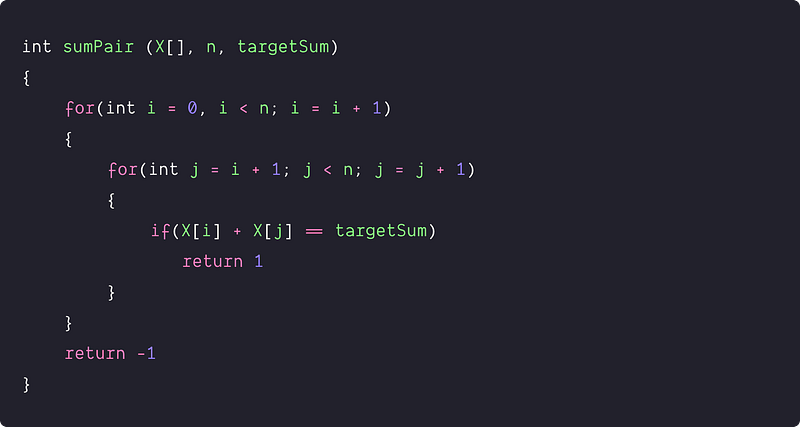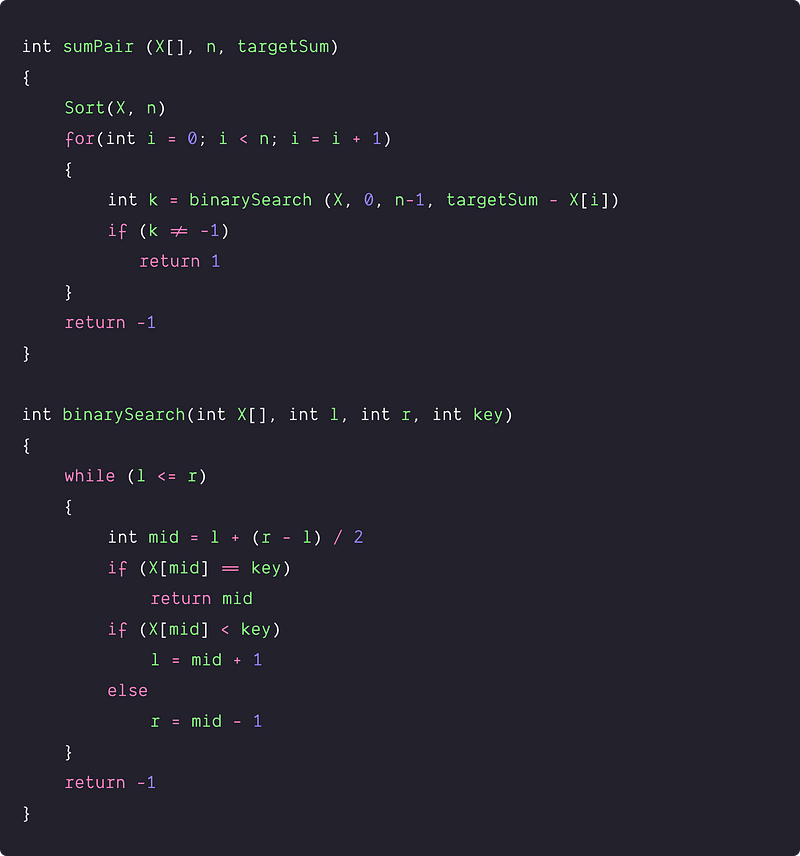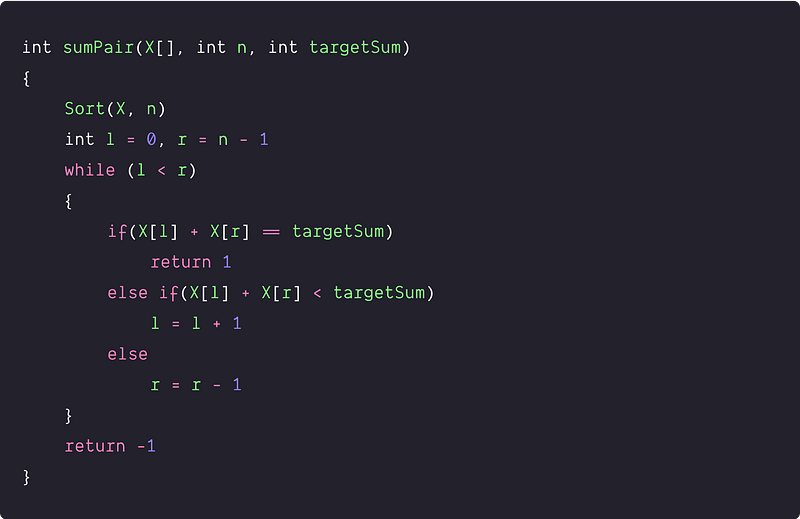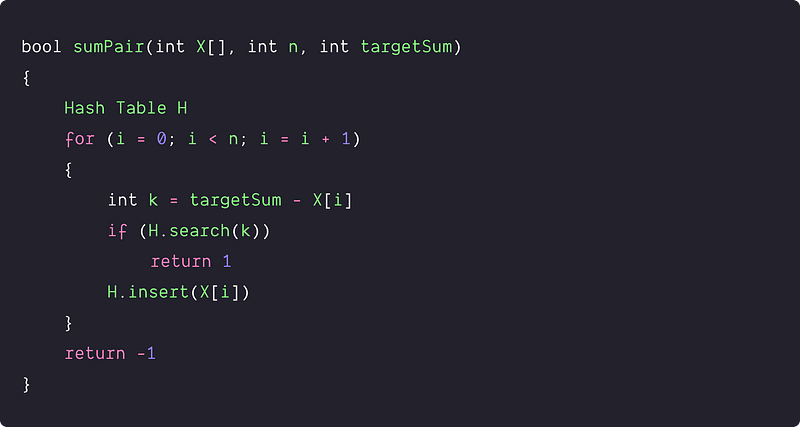# Check for pair in an array with a given sum

Key takeaway

• A famous searching problem can be solved using various approaches.
• The two-pointers and hash table solution are worth exploring.
• One can find variations of this problem asked during a coding interview.

### Let’s understand the problem

Given an array of n integers and given a number targetSum, write a program to determines whether there is a pair of elements in the array that sums to exactly targetSum.

• We assume all elements are distinct.
• Value in the array can be both negative and positive.

Example 1

Input: X[] = [-5, 1, -40, 20, 6, 8, 7], targetSum = 15, Output: true

Explanation: (7,8) or (-5, 20) are the pairs with sum 15.

Example 2

Input: X[] = [-5, 4, -2, 16, 8, 9], targetSum = 15, Output: false

Explanation: There is no pair of elements whose sum is equal to 15.

Important note: Before moving to the solutions, we recommend learners solve this problem. If solved, then well done! We would like to hear your ideas in the comment. Otherwise, no problem, this is an opportunity to learn a new pattern in problem-solving!

### Discussed solution approaches

• A brute force approach  using nested loops
• Using sorting and binary search
• Using sorting and two pointers approach
• An efficient approach  using a hash table

### A brute force approach  using nested loops

A simple idea would be to use two nested loops and check each pair (i, j) in X[]. If there exists a pair with a sum equals to targetSum then we return true otherwise, By end of both loops if we didn’t find such a pair then we return false.

Solution PseudocodeTime and space complexity analysis

We are exploring all possible pairs in the array and doing constant operations for each pair. Total no. of possible pairs = nC2 = n(n-1)/2 = O(n²). So, Time Complexity = O(n²). we are using constant extra space, Space Complexity = O(1). But the critical question  is— how can we improve the time complexity further?

### Using sorting and binary search

Solution Idea

Another idea would be  to sort the array and for every value X[i], apply binary search to find value targetSum - X[i] in the array. Binary search performs searching in O(logn) which could help us to improve the time complexity. If targetSum - X[i] is present then return 1 otherwise return false.

Solution Steps

• Sort the array X[] in increasing order
• Run a loop for each element X[i], apply binary search to look for targetSum - X[i].
• If there exists a value targetSum - X[i] in the array, then return true.
• If there is no such a pair in the whole array, then return false.

Solution PseudocodeTime and space complexity analysis

Suppose we are using efficient O(nlogn) sorting algorithm heap sort and implementing binary search iteratively to search targetSum - X[i] n times.

Time Complexity = Time complexity of heap sort + n* Time complexity of binary search = O(nlogn) + n. O(logn) = O(nlogn) + O(nlogn) = O(nlogn)

Space Complexity = Space Complexity of heap sort + Space complexity of the iterative binary search = O(1) + O(1) = O(1)

### Using sorting and two-pointer approach

Solution Idea

Can we optimize the above approach and find a different method to search the pair in the sorted array? Can we apply two pointer approach because sometimes it works perfectly in the sorted array? Let’s think!

Sort the array and then walk two pointers inward from both ends of the array, at each point looking at their sum. If the sum of the pair is equal to targetSum, then we are done and return true. But if the sum exceeds the value of targetSum, then any sum using the larger element is too large, so we move the right pointer inwards towards a smaller sum. If the sum is less than targetSum, then any sum using the lower element is too small, so we move the left pointer inwards towards a larger sum. By the end of this process, if both pointers cross each other, the pair is not present in the array, and we return false.

Solution Steps

1. Sort the input array in increasing order
2. Now we initialize two pointers in the sorted array, left pointer l = 0, and right pointer r = n-1
3. Run a loop while l < r
4. If (X[l] + X[r] == targetSum) then return true
5. if( X[l] + X[r] < targetSum) then increment l by 1
6. if( X[l] + X[r] > targetSum) then decrement r by 1
7. If didn’t find such a pair in the whole array — return false.

Solution PseudocodeTime and space complexity analysis

Suppose we are using in-place O(nlogn) sorting algorithm heap sort. But now the critical question is — what would be the time complexity of the while loop of two pointer approach?

On the basis of comparison, we are moving either left or right pointer by 1. In the worst case, we are accessing each value of the array using two pointers. So time complexity of two pointers approach = O(n)

Overall time complexity = Time complexity of the heap sort + Time complexity of the while loop = O (n log n) + O(n) = O (n log n). Space Complexity = O(1), we using constant extra space.

### An efficient approach  using a hash table

Solution Idea

We improved the time complexity in the last two solutions, but it is still in the range of O(nlogn) due to the dominance of the sorting algorithm. So now the critical question — how can we optimize further to solve it using linear time complexity? If we observe the above solutions closely, searching is the critical operation for the solution. So the best idea is to use the Hash Table to improve the time complexity further because it performs searching efficiently in the O(1) average.

So the idea would be to iterate over the array and insert element X[i] into the Hash table. Before inserting X[i], we check if the targetSum - X[i] already exists in the table. If it exists, we have found a pair with a sum equal to the targetSum and we return true.

Solution Steps

• Take a Hash Table of size equal to n
• Run a loop and scan over the array X[] for each X[i]. Check if targetSum - X[i] is present in the hash table or not. If yes, we have found the pair and return it true. If no, then insert X[i] into the Hash table.
• If we didn’t find such a pair by end of the loop then return false.

Solution PseudocodeTime and space complexity analysis

In the worst-case, we scan the whole array and didn’t find any such pair. Searching and insertion in the hash table are the critical operations.

Time complexity = Time complexity of inserting n elements into the hash table + Time complexity of searching targetSum - X[i] n times into the hash table = n. O(1) + n . O(1) = O(n) + O(n) = O(n).

Space complexity = O(n), we are using a hash table of size O(n).

Important Note: We recommend learners transform the above pseudo-codes into a favorite programming language (C, C++, Java, Python, etc.) and verify all the test cases. Enjoy programming!

### Critical ideas to think!

• Is there any other way to solve this problem? Think!
• In the last approach, what would be the time and space complexity if we use a BST in place of a hash table?
• In the 2nd and 3rd approaches, what would be the time and space complexity if we use quicksort?
• Is all the above algorithm works fine if elements are repeated?
• Why the idea of the two-pointers approach works perfectly for a sorted array? Provide Proof of the correctness of the two-pointers approach.
• In all the above approaches, do we need to handle the case for the negative values separately?
• How do we modify the above code if we need to return a pair with the targetSum?
• How do we modify the above code if we need to count all pairs with the targetSum?

### Comparisons of time and space complexities

• Two nested loops: Time = O(n^2), Space = O(1)
• Sorting and binary search: Time = O(nlogn), Space = O(1)
• Sorting and Two Pointers: Time = O(nlogn), Space = O(1)
• Hash Table: Time = O(n), Space = O(n)

### Similar coding questions to practice

Enjoy learning, Enjoy coding, Enjoy algorithms!

Get well-designed application and interview centirc content on ds-algorithms, machine learning, system design and oops. Content will be delivered weekly.